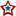#MN-Sen: Recount Regression, Day 8

For a more complete explanation of the process used, please refer to my initial post on the subject.

Recap of today's official result:

```            Recount         Original
Coleman:   1,044,255   -   1,046,101  =  -1,846
Franken:   1,040,285   -   1,042,208  =  -1,923

Franken Net Today: -100 (-20 Yesterday, -5 day before, 14)
Total Franken Net:  -77
Franken Deficit:    292
```

The following two graphs depict the number of votes gained or lost with respect to the total votes recorded for each precinct that has completed their recount. Each dot represents the change between the originally certified result and the post recount tally in a given precinct with x number of total votes:And the current functions used in conjunction with the regression:Now again using those functions, the following two graphs further illustrate the regression interpolation. The graph below illustrates the regression on precincts whose vote totals are less than 3,858; the largest precinct that has completed a recount. The dotted lines represent the two-day-ago regression, while the dashed lines represent yesterday's. The thin lines depict the linear regression while the thicker curvy lines present a quartic regression. The black bars emanating from the x axis represent the number of precincts, statewide with x number of total votes. There are a few straggler precincts between 2600 and 6621 that are not depicted due to the resolution of the graph.The graph below goes further and fits the regression onto all 4,130 precincts statewide; this forces 17,270 additional votes, that lie in precincts that surpass the 3,858 vote threshold, into the predetermined trend.Using the previous two graphs, and the functions they represent, a projection can be made for the cases covered. The first case simply includes precincts with 3,858 or less, while precincts above and beyond that figure are entirely ignored. For each precinct, the total vote total is taken and applied to the listed function for each candidate. The result is then added to that candidate's sum, and the next precinct is calculated. This process is done using precinct results from the final certification.

```            Coleman: -1599 (+/- 92)
Franken: -1612 (+/- 95)

Net Franken: -83.169 (+/- 187)
```

Franken is now actually projected to lose votes, about 83 to be exact but with all the challenges outstanding, these projections are far from accurate. There are also 17,270 votes excluded from the above extrapolation. If those additional 17,270 votes are applied to the process, nothing really changes but the margin of error:

```            Coleman: 1610- (+/- 236)
Franken: 1623- (+/- 244)

Net Franken: -83.703 (+/- 580)
```

The graph below shows the number of challenges by each candidate relative to how they are performing in a given precinct. The dots represent challenges; a dot to the north of the x-axis represents a precinct that the candidate is currently winning. A dot to the south shows the number of challenges in a precinct that each candidate is currently losing. There are no negative challenges.The next graph shows each candidate's challenge regression on the same graph:It appears that a larger percent of Franken challenges occur in precincts he is currently winning, relative to Coleman's percentage. They both appear to be challenging more ballots in precincts they are winning, but Franken at a higher rate. This discrepancy may allow Franken to make up additional votes, but an exact number is impossible to predict.

This analysis is valid as of right now (10:22 PM CT 11/26), after 89.64% of all votes have been recounted. Recounting should resume on the first of December after a Thanksgiving recess.

## 0 Response(s) to MN-Sen: Recount Regression, Day 8(Word Verification)